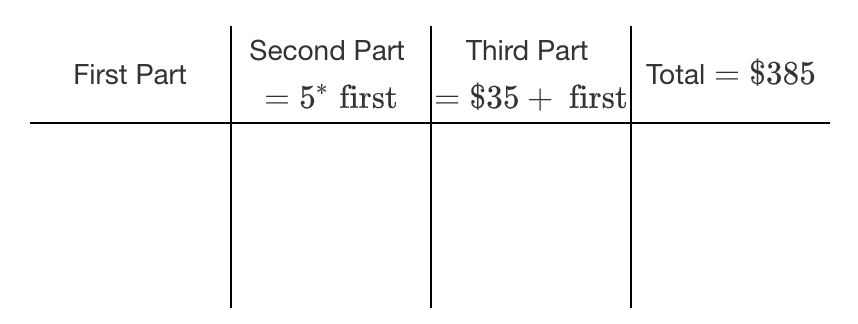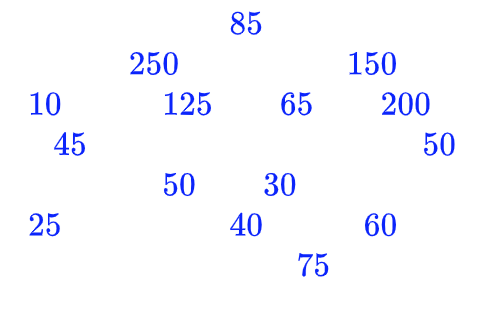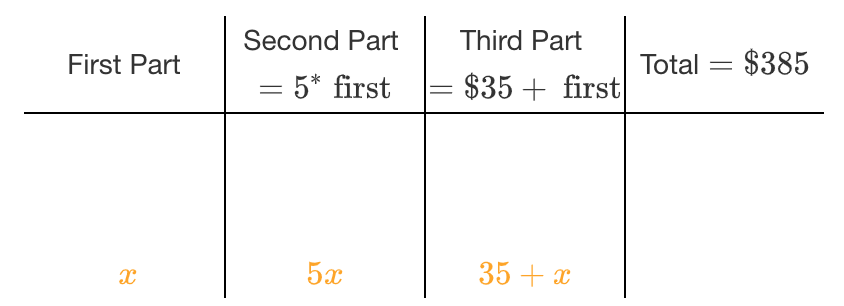### Home > CC3MN > Chapter 3 > Lesson 3.1.1 > Problem3-6

3-6.

Ms. Nguyen needs to separate $\385$ into three parts to pay some debts. The second part must be five times as large as the first part. The third part must be $\35$ more than the first part. How much money must be in each part? Write and solve an equation.

You can use 5-D Process to help you write the equation. A row of 4 columns, labeled from left to right, as follows: First Part. Second Part, = 5, times first. Third Part, = $35, + first. Total =$385.

Use $2$ or $3$ of the blue numbers at the right as guesses for the first part and calculate the second and third part. Randomly placed numbers: 85, 150, 250, 65, 200, 10, 125, 45, 50, 30, 50, 25, 40, 60, 75.

Use the expressions shown in the table above and your trials in the table to write an equation. Solve your equation. Does your answer make sense? Second row added to the table, labeled from left to right, as follows: x, 5 x, 35 + x, blank.